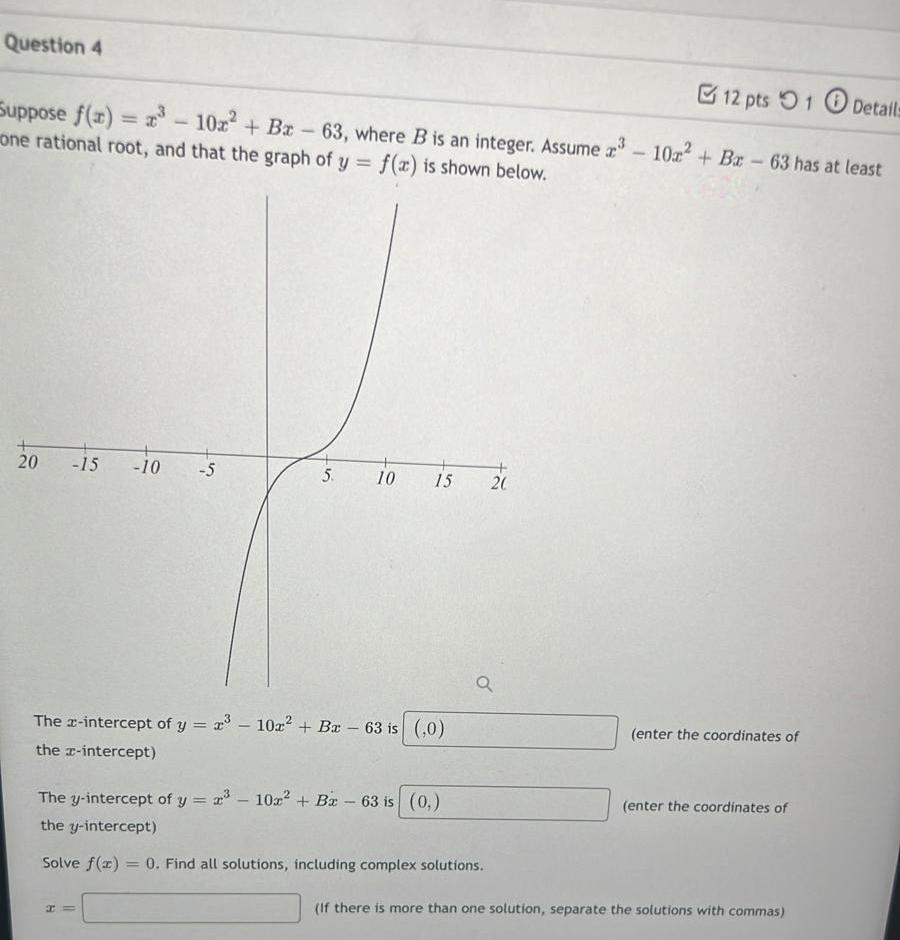Question:

# Question 4 12 pts 1 Details Suppose f(u) = ?? - 102+ Bx - 63, where B is an integer. Assume 23 - 10x² + Bc - 63 has at least x oQuestion 4 12 pts 1 Details Suppose f(u) = ?? - 102+ Bx - 63, where B is an integer. Assume 23 - 10x² + Bc - 63 has at least x one rational root, and that the graph of y = f(x) is shown below. 20 -15 -10 -5 5 10 15 + 20 Q The c-intercept of y = 23 – 10x² + Bx - 63 is (,0) the I-intercept) (enter the coordinates of The y-intercept of y= 2:3 - 102? + Bx - 63 is (0) the y-intercept) (enter the coordinates of Solve f(3) 0. Find all solutions, including complex solutions. (If there is more than one solution, separate the solutions with commas)